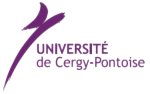5ECM Minisymposium on Random and Quasiperiodic Operators.
Thursday July 17, 2008, 13:25 - 14:55 + 15:25 - 16:55

 In solid states physics, one of the main problems in the study of disordered media is to describe electronic transport. This problem is quantum in nature. The Schrödinger equation is thus the natural mathematical model for these problems. A macroscopical sample of a disordered medium, such as an alloy or a semi- conductor contains a vast number of particles. Thus, the rigorous mathematical study of the full quantum model is intractable. Nevertheless, in many cases, simplifying assumptions and approximations can be physically justified. Two very important models thus obtained are random and quasi-periodic Schrödinger operators. The last 20 years have seen the development of a very active field creating a vast literature on these topics. Many different branches of mathematics have been directly concerned with this topic, e.g. partial differential equations, spectral theory, probability theory, dynamical systems, mathematical physics, etc. The goal of this mini-symposium is to give its audience an overview over recently accomplished progress in this field covering many of the different areas of mathematics that enabled this progress.
Invited Speakers:

Alexander Fedotov University of Saint Petersburg, Saint-Petersburg, Russia
 On the behavior at inﬁnity of solutions of an almost periodic equation. We study the behavior at inﬁnity of solutions of a model almost periodic equation with a ”large” coupling constant using the monodromization renormalization method. In the course of the renormalizations, one obtain an inﬁnite series of almost periodic equations describing a given solution of the input equation subsequently on larger and larger intervals Il, l = 1,2,3…, the ratio of their length being determined by the continued fraction for the ratio of the periods of the input operator. This allows to control the solutions of the input equation in a constructive way. For a given value of the ergodic parameter, the behavior of the solutions drastically depends on the existence of the Lyapunov exponent. Roughly, when the Lyapunov exponent exists there are solutions that are nicely exponentially increasing, and when it does not exists, there is an inﬁnite subsequence of the above intervals such that, on each of the intervals Ilk of this subsequence, ( if we ”forget” about the details of the behavior of the solutions on the distances of order of the length of Ilk-1, Ilk-2 etc ) the solution ﬁrst increases and then begins to decay. The results were obtained in collaboration with F. Klopp (Univ. Paris 13).

Abel Klein University of California at Irvine, Irvine, CA, USA
 Poisson Statistics for Eigenvalues of Continuum Random Schrödinger Operators. We prove Poisson statistics for eigenvalues of random Schr\"odinger operators in the continuum. More specifically, we prove a Minami estimate for continuum Anderson Hamiltonians in the continuum and derive Poisson statistics for the eigenvalues in the localization region at the bottom of the spectrum. We also prove simplicity of the eigenvalues in that region. (Joint work with J.-M. Combes and F. Germinet)

Wolfgang König Universität Leipzig, Leipzig, Germany
 A two-cities theorem for the parabolic Anderson model. The parabolic Anderson problem is the Cauchy problem for the heat equation ∂tu(t,z) = Δu(t,z)+ξ(z)u(t,z) on (0,∞) × Zd with random potential (ξ(z): z ∈ Zd). We consider independent and identically distributed potentials, such that the distribution function of ξ(z) converges polynomially at inﬁnity, i.e., a heavy-tailed distribution. If u is initially localised in the origin, i.e., if u(0,x) = δ0(x), we show that, as time goes to inﬁnity, the solution is completely localised in two points almost surely and in one point with high probability. We also identify the asymptotic behaviour of the concentration sites in terms of a weak limit theorem. This is joint work with H. Lacoin, P. Mörters, and N. Sidorova.

Raphael Krikorian Université Paris 6, Paris, France
 KAM-Liouville Theory and an extension of a theorem by Dinaburg and Sinai. We prove that given any irrational frequency α on the one dimensional torus T, the Schrödinger cocycle associated to an analytic potential on T above the rotation by α, is conjugated to an SO(2,R)-valued cocycle (and hence bounded) provided the potential is small enough and the rotation number of the cocycle satisﬁes a diophantine condition w.r.t. α. When α is ﬁxed, the theorem holds for a set of positive measure of the energy. This is an extension of a theorem of Dinaburg and Sinai to the case where α is not diophantine. The technique of the proof is based on a perturbative scheme, reminiscent of KAM theory but that applies for any α irrational. This is a joint work with Artur Avila and Bassam Fayad.

Armen Shirikyan Université de Cergy-Pontoise, Cergy-Pontoise, France
 Control and mixing for nonlinear PDE's. Let us consider a differential equation subject to an external force. It is well known that, in the finite-dimensional case, the controllability of the equation in question and the mixing property of the associated random dynamics are essentially equivalent. The situation is much more delicate in the infinite-dimensional setting. In this talk, I give an overview of some recent results on the problem of controllability and mixing for a class of PDE's arising in mathematical physics and formulate some open questions.

Simone Warzel Princeton University, Princeton, NJ, USA.
 On the Joint Distribution of Energy Levels of Random Schroedinger Operators. We consider operators with random potentials on graphs, such as the lattice version of the random Schroedinger operator. The main result is a general bound on the probabilities of simultaneous occurrence of eigenvalues in specified distinct intervals, with the corresponding eigenfunctions being separately localized within prescribed regions. The bound generalizes the the Wegner estimate on the density of states. The analysis proceeds through a new multiparameter spectral averaging principle.
 Organizers: Sponsors: François Germinet, Université de Cergy-Pontoise Frédéric Klopp, Université Paris-Nord